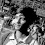## Proof of Cauchy-Schwarz in R^n

If ${\bf x} = (x_1, x_2, \dotsc , x_n), {\bf y} = (y_1, y_2, \dotsc, y_n) \in \Bbb{R}^{n}$, i.e. the $x_{i}$'s and $y_{i}$'s are all real numbers, then the Cauchy-Schwarz inequality states that: $$\left( \sum_{i=1}^{n}{x_{i} y_{i}} \right)^{2} \leq \left( \sum_{i=1}^{n}{x_{i}^{2}} \right) \left( \sum_{i=1}^{n}{y_{i}^{2}} \right)$$ This is actually a special case of the more general Cauchy-Schwarz inequality, but this post is just to show a neat proof (straight from Wikipedia) of this special case, which will be used in a few other posts. You'll need to recall quadratics and discriminants from algebra for this proof.

Proof of Cauchy-Schwarz for $\Bbb{R}^{n}$: If all the $x_i$'s or all the $y_i$'s are zero, then both sides of the inequality are zero, and the inequality is trivially true. So assume that at least one of the $x_i$'s and at least one of the $y_i$'s are non-zero. Regard the $x_{i}$'s and $y_{i}$'s as fixed for a moment, and consider the following quadratic polynomial in the variable $z$ : \begin{align} p(z) &= (x_1 z + y_1)^{2} + (x_2 z + y_2)^{2} + \dotsb + (x_n z + y_n)^{2} \\[3mm] &= \underbrace{\left( \sum_{i=1}^{n}{x_{i}^{2}} \right)}_{a} z^2 + \underbrace{\left( 2 \sum_{i=1}^{n}{x_{i} y_{i}} \right) }_{b} z + \underbrace{\left( \sum_{i=1}^{n}{y_{i}^{2}} \right) }_{c} \end{align} From the first formula, $p(z)$ is the sum of squares, so we know that $p(z) \geq 0$ for any value of $z$. Furthermore, since $a$ is the sum of squares, we also have $a > 0$ (strictly greater, since at least one of the $x_i$'s is non-zero), which means the graph of $p(z)$ is an upward-opening (i.e. "holds water") parabola.

These two facts combined mean that $p(z)$ has at most one real root, and so the discriminant $b^2 - 4ac \leq 0$. Thus $$\left( 2 \sum_{i=1}^{n}{x_{i} y_{i}} \right)^2 - 4 \left( \sum_{i=1}^{n}{x_{i}^{2}} \right) \left( \sum_{i=1}^{n}{y_{i}^{2}} \right) \leq 0 \\[3mm] \Downarrow \\[3mm] \left( \sum_{i=1}^{n}{x_{i} y_{i}} \right)^2 \leq \left( \sum_{i=1}^{n}{x_{i}^{2}} \right) \left( \sum_{i=1}^{n}{y_{i}^{2}} \right)$$ $\square$

1.Note that in the diagram, the horizontal and vertical axes should be labeled $z$ and $p(z)$ respectively, and the equation displayed would be $p(z) = az^{2} + bz + c$. I was just too lazy to go into MS Paint and make the changes :)
2.3.